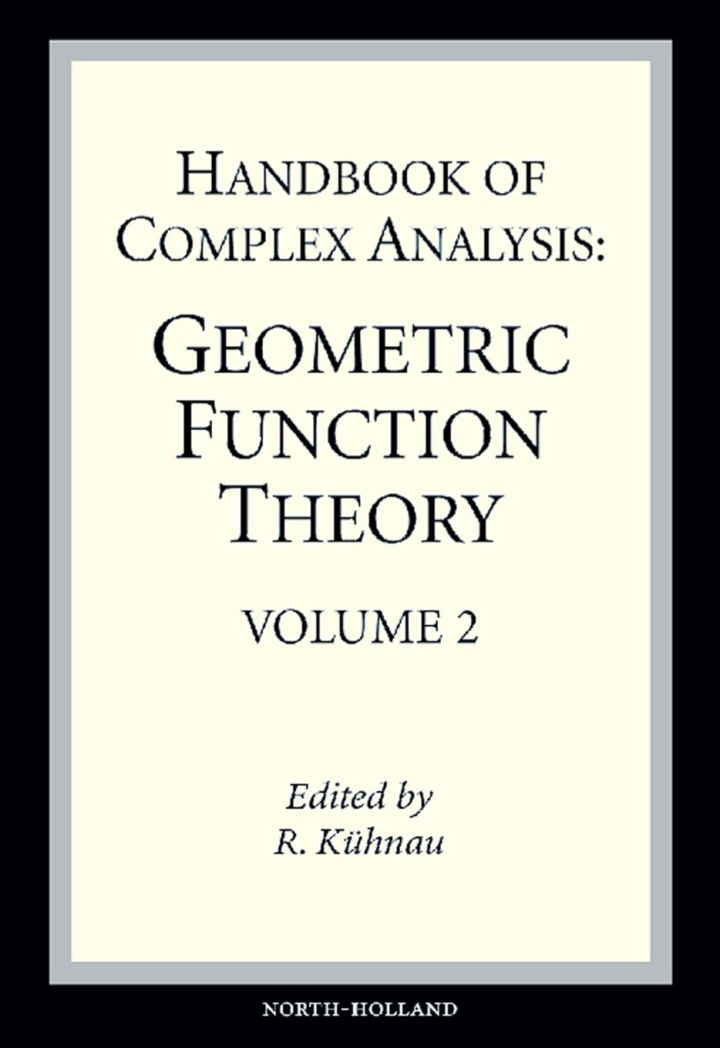# Handbook of Complex Analysis: Geometric Function Theory

## Handbook of Complex Analysis: Geometric Function Theory

By Kuhnau, Reiner

### Rent or Buy eTextbook

Expires on Apr 14th, 2022
\$305

Publisher List Price: \$329.99
Savings: \$24.99

Geometric Function Theory is that part of Complex Analysis which covers the theory of conformal and quasiconformal mappings.

Beginning with the classical Riemann mapping theorem, there is a lot of existence theorems for canonical conformal mappings. On the other side there is an extensive theory of qualitative properties of conformal and quasiconformal mappings, concerning mainly a prior estimates, so called distortion theorems (including the Bieberbach conjecture with the proof of the Branges). Here a starting point was the classical Scharz lemma, and then Koebe's distortion theorem.

There are several connections to mathematical physics, because of the relations to potential theory (in the plane). The Handbook of Geometric Function Theory contains also an article about constructive methods and further a Bibliography including applications eg: to electroxtatic problems, heat conduction, potential flows (in the plane).

· A collection of independent survey articles in the field of GeometricFunction Theory
· Existence theorems and qualitative properties of conformal and quasiconformal mappings
· A bibliography, including many hints to applications in electrostatics, heat conduction, potential flows (in the plane).

Subject: Mathematics & Statistics -> Mathematics -> Mathematics GeneralPublisher: Elsevier S & T 12/2004
Imprint: North Holland
Language: English
Length: 876 pages

ISBN 10: 044451547X
ISBN 13: 9780444515476
Print ISBN: 9780444515476

Live Chats